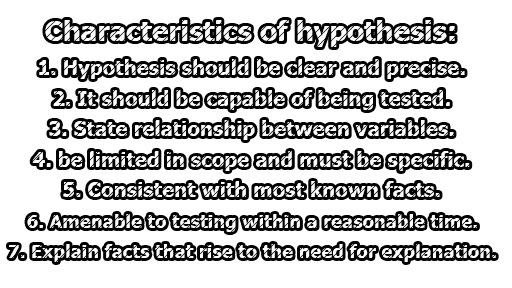## Characteristics of hypothesis

A hypothesis is usually considered the principal instrument in research. Its main function is to suggest new experiments and observations. In fact, many experiments are carried out with the deliberate object of testing hypotheses. Decision-makers often face situations wherein they are interested in testing hypotheses on the basis of available information and then take decisions on the basis of such testing. A researcher’s hypothesis is a formal question that he intends to resolve. Some of the characteristics of the hypothesis are being:Figure: Characteristics of hypothesis
1. Hypothesis should be clear and precise. If the hypothesis is not clear and precise, the inferences drawn on its basis cannot be taken as reliable.
2. Hypothesis should be capable of being tested. In a swamp of untestable hypotheses, many a time the research programs have bogged down. Some prior studies may be done by researchers in order to make the hypothesis a testable one. A hypothesis “is testable if other deductions can be made from it which, in turn, can be confirmed or disproved by observation.”
3. Hypothesis should state the relationship between variables if it happens to be a relational hypothesis.
4. Hypothesis should be limited in scope and must be specific. A researcher must remember that narrower hypotheses are generally more testable and he should develop such hypotheses.
5. Hypothesis should be stated as far as possible in most simple terms so that the same is easily understandable by all concerned. But one must remember that simplicity of the hypothesis has nothing to do with its significance.
6. Hypothesis should be consistent with most known facts i.e., it must be consistent with a substantial body of established facts. In other words, it should be one which judges accept as being the most likely.
7. Hypothesis should be amenable to testing within a reasonable time. One should not use even an excellent hypothesis, if the same cannot be tested in a reasonable time for one cannot spend a lifetime collecting data to test it.
8. Hypothesis must explain the facts that gave rise to the need for explanation. This means that by using the hypothesis plus other known and accepted generalizations, one should be able to deduce the original problem condition. Thus hypothesis must actually explain what it claims to explain; it should have the empirical reference.

References: Research Methodology Methods and Techniques by C.R. Kothari